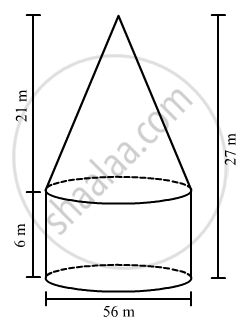Advertisement Remove all ads

# A Circus Tent is in the Shape of Cylinder Surmounted by a Conical Top of Same Diameter. If Their Common Diameter is 56 M, the Height of the Cylindrical Part is 6 M and the Total Height - Mathematics

Answer in Brief

A circus tent is in the shape of cylinder surmounted by a conical top of same diameter. If their common diameter is 56 m, the height of the cylindrical part is 6 m and the total height of the tent above the ground is 27 m, find the area of the canvas used in making the tent.

Advertisement Remove all ads

#### SolutionTotal height of the tent above the ground = 27 m
Height of the cylinderical part,

$h_1$= 6 m
Height of the conical part,

$h_2$ = 21 m
Diameter = 56 m
Radius = 28 m
Curved surface area of the cylinder, CSA1 = $2\pi r h_1 = 2\pi \times 28 \times 6 = 336\pi$

Curved surface area of the cylinder, CSA2 will be

$\pi rl = \pi r\left( \sqrt{h^2 + r^2} \right) = \pi \times 28 \times \left( \sqrt{{21}^2 + {28}^2} \right) = 28\pi\left( \sqrt{441 + 784} \right)$

$= 28\pi \times 35$

$= 980\pi$

Total curved surface area = CSA of cylinder + CSA of cone
= CSA+ CSA2

$= 336\pi + 980\pi$

$= 1316\pi$

$= 4136 m^2$

Thus, the area of the canvas used in making the tent is 4136 m2.

Is there an error in this question or solution?
Advertisement Remove all ads

#### APPEARS IN

RD Sharma Class 10 Maths
Chapter 14 Surface Areas and Volumes
Exercise 14.2 | Q 38 | Page 63
Advertisement Remove all ads
Advertisement Remove all ads
Share
Notifications

View all notifications

Forgot password?